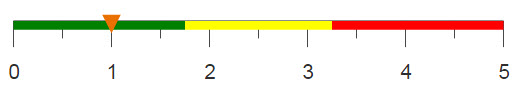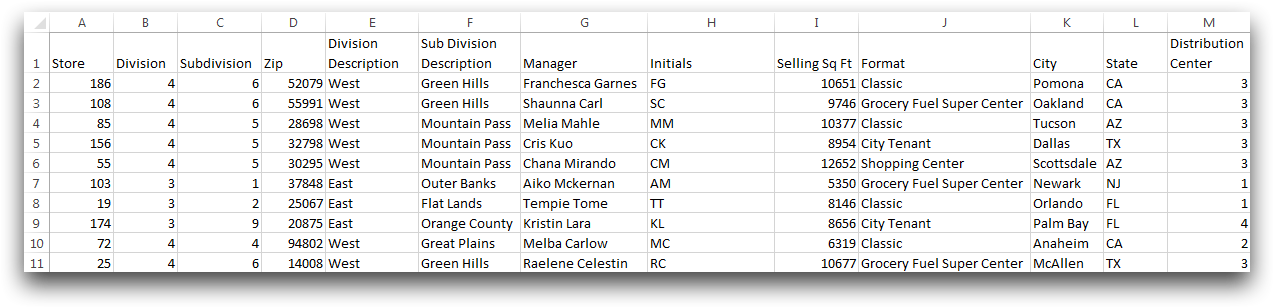# Splitting and concatenating strings

Using string manipulations can convert a column containing names to a column containing initials.

## Difficulty## Objective

You have a column containing the first and last name of a group of people. Because you do not require the full name of each person, you would like to create a column containing just the first and last initial of each person. You know how to do this using the LEFT, RIGHT, and MID functions in Excel, and you would like to achieve the same results using 1010data.

## Excel solution

The LEFT, RIGHT, and MID functions in Excel extract characters from a specified string based on given inputs. To extract the initials from a first and last name contained in the same column, you need to use a combination of all three functions.

Obtaining the first initial is done by using the LEFT function and selecting only the first character starting on the left hand side of the string The second initial is more difficult to obtain. First, the FIND function is used to find the position of the space that separates the first and last name, then MID is used to extract only the space and the character that follows the space. Finally, you can use the RIGHT function to choose only the first character, starting on the right end of the string.

`=LEFT(G2,1)&RIGHT(MID(G2,FIND(" ",G2,1),2),1)`While this combination of functions is sufficient in obtaining the initials, 1010data offers a larger range of string manipulation functions to perform similar tasks.

## 1010data Macro Language solution

```<base table="pub.doc.retail.altseg.stores"/>
<willbe name="first" value="taketo(manager;' ')"/>
<willbe name="second" value="dropto(manager;' ')"/>
<willbe name="first_initial" value="first(first;1)"/>
<willbe name="second_initial" value="first(second;1)"/>
<willbe name="initials" label="Initials"
value="splice(first_initial second_initial;'')"/>
<colord hide="first,second,first_initial,second_initial"/>```

When it is not necessary to retain a complete string within a column, converting to initials can be useful and simplify your data table. In this solution we utilize several string functions to extract the first letter from each name and create a column containing just the initials.

Four columns are created to separate the first and last names, and then to select the leading letter in each name. Then, the splice function is used to combine the individual letters and create the "Initials" column. Using colord you can hide the columns you created to aid in your transformation.

## Alternate 1010data Macro Language solution

```<base table="pub.doc.retail.altseg.stores"/>
<willbe name="initials" label="Initials"
value="splice(first(manager;1) first(dropto(manager;' ');1);'')"/>```

The alternate solution uses the same functions but combines them into one line to simplify the code and avoid the need to create unnecessary columns. This solution also avoids using taketo by simply using the first letter in the name column.

## Further reading

If you would like to learn more about the functions and operations discussed in this recipe, click on the links below:

taketo(X;Y)

dropto(X;Y)

splice(X;Y)